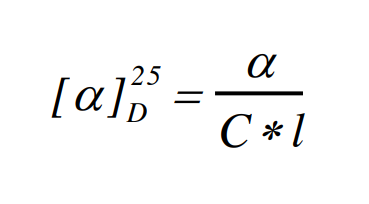# 3.1: Analysis and Discussion

$$\newcommand{\vecs}{\overset { \rightharpoonup} {\mathbf{#1}} }$$ $$\newcommand{\vecd}{\overset{-\!-\!\rightharpoonup}{\vphantom{a}\smash {#1}}}$$$$\newcommand{\id}{\mathrm{id}}$$ $$\newcommand{\Span}{\mathrm{span}}$$ $$\newcommand{\kernel}{\mathrm{null}\,}$$ $$\newcommand{\range}{\mathrm{range}\,}$$ $$\newcommand{\RealPart}{\mathrm{Re}}$$ $$\newcommand{\ImaginaryPart}{\mathrm{Im}}$$ $$\newcommand{\Argument}{\mathrm{Arg}}$$ $$\newcommand{\norm}{\| #1 \|}$$ $$\newcommand{\inner}{\langle #1, #2 \rangle}$$ $$\newcommand{\Span}{\mathrm{span}}$$ $$\newcommand{\id}{\mathrm{id}}$$ $$\newcommand{\Span}{\mathrm{span}}$$ $$\newcommand{\kernel}{\mathrm{null}\,}$$ $$\newcommand{\range}{\mathrm{range}\,}$$ $$\newcommand{\RealPart}{\mathrm{Re}}$$ $$\newcommand{\ImaginaryPart}{\mathrm{Im}}$$ $$\newcommand{\Argument}{\mathrm{Arg}}$$ $$\newcommand{\norm}{\| #1 \|}$$ $$\newcommand{\inner}{\langle #1, #2 \rangle}$$ $$\newcommand{\Span}{\mathrm{span}}$$$$\newcommand{\AA}{\unicode[.8,0]{x212B}}$$

1. Using the refractive index data determines the approximate composition of your oil, your carvone fraction and your limonene fraction with respect to limonene and carvone.
2. From the gas chromatography, report the percentages of limonene and carvone in your original sample and in the fractions following distillation. How effective was the distillation in separating these components? Are there any other (minor) components in any of the fractions? If so, how much of the sample do they constitute? Can you identify any of the minor components? Calculate the # of theoretical plates for each sample peak n = 5.55 (tr/w1/2)2
3. Compare the results obtained from gas chromatography and refractive index measurements. How closely do they agree? If they are not in good agreement can you provide an explanation?
4. Which isomer of carvone do you have? Is it R or S? How do you know? What is the association between the chirality and the odor of the carvone isomers?
5. Calculate the specific rotation of the semicarbazone derived from carvone,where α is the rotation angle read on the polarimeter, C is the concentration of the semicarbazone solution in grams per milliliter of solution, and l is the path length of the polarimeter tube in decimeters (these units are employed for historical, rather than rational reasons). Include a propagation of errors.

6. (a) Which features in the IR spectrum, can be assigned to limonene? To carvone? Identify as many functional groups as you can in each sample.

(b) Do the spectra of the samples suggest the presence of a mixture of these two components, and if so, are they consistent with the GC data?

(c) Can you use IR spectroscopy to distinguish between (R)- carvone and (S)- carvone?

Your well written discussion should address the following (within the text, NOT as a numerical series of answers to questions):

1. Comparison of your distillation results (temperature ranges) with your chromatographic results.
2. Explanation of how the separation of components could be improved.
3. Comparison of your results (boiling points, compositions, m.p. of semicarbazones, optical rotations) with literature values.
4. Discussion of what information gas chromatography provides.
5. Discussion of what information infrared spectroscopy provides.
6. Discussion of what information X-Ray Crystallography provides
7. Discussion of what information these techniques do NOT provide.
8. Discussion of what the identity of the carvone enantiomer tells you (if anything) about the identity of the limonene in your sample.